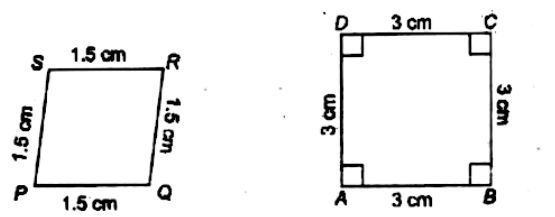Home/Class 10 Maths Chapter List/4. Triangles/

# State whether the following quadrilaterals are similar or not.

State whether the following quadrilaterals are similar or not.Speed
00:00
02:17## QuestionMathsClass 10

State whether the following quadrilaterals are similar or not.see the solution given below
4.64.6## Solution

For two figures/shapes to be similar, their sides should be in proportion and their angles should be equal.The two quadrilaterals, in figure are not similar because their corresponding angles are not equal though their sides are in proportion. It is clear from the figure that $$\angle A$$ is $$90^{o}$$ but$$\angle P$$ is not $$90^{o}$$.
Note: The two shapes are also different, therefore, they cannot be similar as one is a square and other is a rhombus.